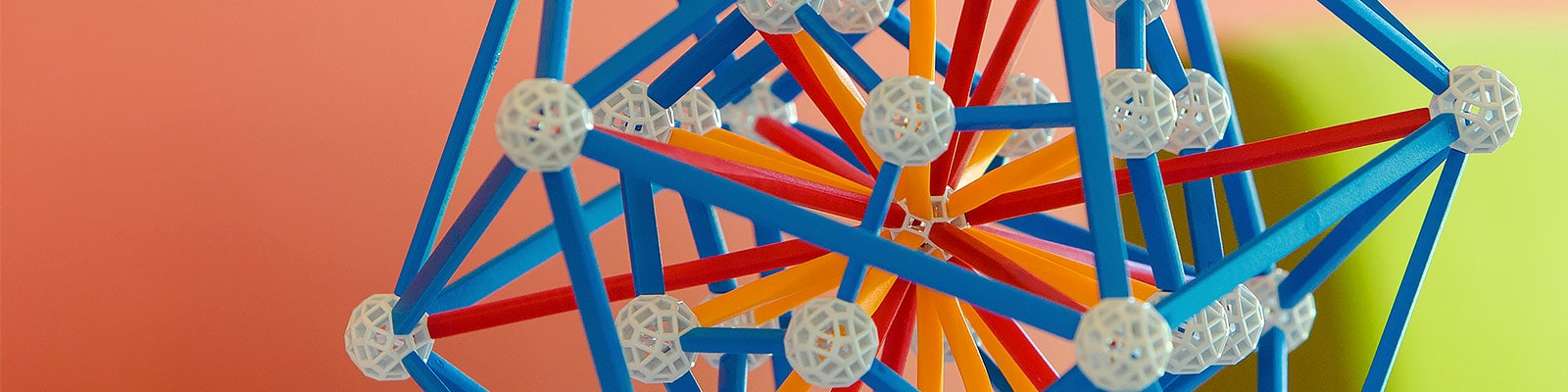# Algebra and Geometry

The Algebra and Geometry group is an integral part of the Pure Mathematics section of the department, with several members also being part of the other two groups. The group conducts research in a wide variety of subjects of algebraic and geometric nature.

## Projects

Singularities and symplectic topology
25/03/2019 → 13/12/2020
Research

Linearly constrained frameworks
01/11/2018 → 31/01/2019
Research

Functor categories for groups
01/10/2017 → 31/12/2018
Other

LMS Scheme 4 - 41434
01/09/2015 → 30/09/2015
Other

Reconstructing Broken Symmetry
28/11/2014 → 27/03/2016
Research

EPSRC - Interpretation functors and infinite-dimensional representations of finite-dimensional algebras
09/09/2013 → 08/09/2016
Research

## Research Areas

### Homotopical and homological algebra

This subject is associated with the names of MacLane, Eilenberg, Cartan and later Quillen; it has its origins in topology, however it has by now become a standard tool in representation theory, algebraic geometry and even parts of theoretical physics (A.Lazarev, N. Mazza).

### Mathematical physics

The last couple of decades have been punctuated by a steady penetration of methods of mathematical physics into pure mathematics, resulting in breakthrough developments in algebraic and differential geometry. Particularly the philosophy of the Feynman path integral turned out to be especially fruitful in tackling purely mathematical problems (R. Hillier, A. Lazarev).

### Deformation theory

The aim of deformation theory is to analyse the space of objects of an algebraic or geometric nature (i.e. a complex manifold, a coherent sheaf, a Lie algebra, etc.) in the neighbourhood of a given one. Many new and exciting developments resulted from this simple idea, for example Drinfeld's theory of quantum groups and Kontsevich's deformation quantization (J. Grabowski, A. Lazarev, P. Levy).

### Algebraic Topology

Algebraic topology studies geometric shapes and their properties which do not change under continuous deformation (homotopy). It is closely related and provides motivation for, homological and homotopical algebra (A. Lazarev).

### Representation theory of groups and algebras

A lot of information about an algebraic structure such as a group or algebra could be obtained by studying its actions on vector spaces. This is the subject of representation theory; it is closely related to combinatorics and homological algebra (P.Levy, N. Mazza, G. Elek).

### Structure theory of groups and algebras

The notion of a group is one of the simplest yet most profound in the whole of mathematics. Certain classes of these objects, so-called simple finite groups and simple algebras have been classified; however for large classes of groups and Lie algebra the structure theory contains a lot of unanswered questions and is being actively developed (G. Elek, N. Mazza, D. Towers).

### Nonassociative and Lie algebras

The notion of a Lie algebra is an infinitesimal version of that of a group; it is just as ubiquitous and has deep connections to the theory of Lie groups and algebraic groups. While finite-dimensional simple Lie algebras have been classified, other classes of (e.g. nilpotent, soluble) are much less understood. Lie algebras are special cases of nonassociative algebras, among other examples of current interest are Jordan algebras, Leibniz algebras, genetic algebras etc. (M. MacDonald, D. Towers).

### Algebraic groups

An algebraic group is a group in the category of algebraic varieties, which means that it is locally described by a system of polynomial equations. The study of algebraic groups is part of algebraic geometry, having deep connections to Lie groups and Lie algebras (J. Grabowski, P. Levy, M. MacDonald).

### Noncommutative Geometry

Noncommutative geometry looks at noncommutative rings and algebras as if they were rings of functions on certain `noncommutative spaces'. This point of view was pioneered by A. Connes and it has various ramifications in algebra, geometry and even analysis. It is related to deformation theory, operator algebras and several aspects of mathematical physics (J. Grabowski, R. Hillier).

### Geometric Group Theory

Geometric group theory seeks to study finitely generated groups by associating certain topological or metric spaces to them and translating algebraic questions into geometric ones (G. Elek).

### Combinatorics

Combinatorics is a branch of mathematics which studies discrete, usually finite structures such as graphs, polytopes, tilings etc. It has innumerable connections to other fields: group theory, representation theory, ergodic theory, discrete and computational geometry, computer science and many others (G. Elek, T. Nixon, B. Schulze).